×#### Thank you for registering.

One of our academic counsellors will contact you within 1 working day.

Click to Chat

1800-1023-196

+91-120-4616500

CART 0

• 0

MY CART (5)

Use Coupon: CART20 and get 20% off on all online Study Material

ITEM
DETAILS
MRP
DISCOUNT
FINAL PRICE
Total Price: Rs.

There are no items in this cart.
Continue Shopping• Complete JEE Main/Advanced Course and Test Series
• OFFERED PRICE: Rs. 15,900
• View Details

```Revision Notes on Congruence of Triangles

Congruence

If we superpose one figure over other and they fit into each other then they must be congruent shapes. They must have the same shape and size.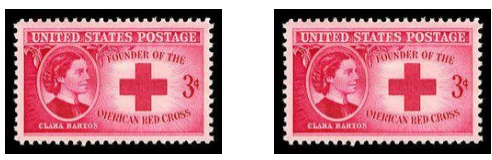Symbol of Congruence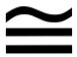Congruence of 2-dimensional Shapes

In the case of 2D shapes, the two shapes will be congruent if they have the same shape and size. You cannot bend, stretch or twist the image.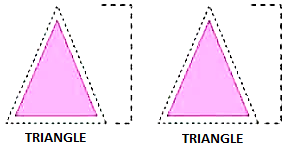Congruence among Line Segments

To check whether the line segments are congruent or not, we can superpose one line segment over another and if they completely cover each other then they must be congruent.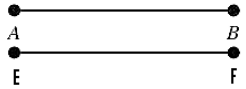Two line segments are congruent if they have equal length and if two line segments have equal length then they must be congruent.

Congruence of Angles

Two angles of the same measurement are congruent and if two angles are congruent then their measurement must be the same.Here, ∠R ≅ ∠Q

Congruence of Triangle

If we superpose one triangle over other triangle and they cover each other properly, then they must be congruent triangles.

In case of congruent triangles-

All the sides of one triangle must be equal to the corresponding sides of another triangle.

All the angles of one triangle must be equal to the corresponding angles of another triangle.

All the vertices of one triangle must be corresponding to the vertices of another triangle.In the above triangles,

If, ∆ABC ≅ ∆FDE then

Corresponding vertices are – ∠A ↔ ∠F, ∠B ↔ ∠D and ∠C ↔ ∠E

Corresponding angles are - ∠A ↔ ∠F, ∠B ↔ ∠D and ∠C ↔ ∠E

Corresponding sides are – AB ↔ FD, BC ↔ DE and AC ↔ FE

Remark: It is the order of the letters in the names of congruent triangles which tells the corresponding relationships between two triangles. If we change it from ∆ABC ≅ ∆FDE to ∆BCA ≅ ∆FDE, then it is not necessary that the two triangles are congruent as it is important that all the corresponding sides, angles and vertices are same.

The Criterion for Congruence of Triangles

1. SSS Criterion(Side-Side-Side)

This criterion says that the two triangles will be congruent if their corresponding sides are equal.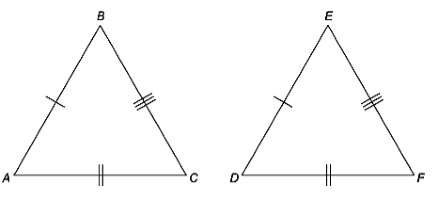If Side AB = DE

Side BC = EF

Side AC = DF

Then, ∆ABC ≅ ∆DEF

Example

In the two given triangles, ∆ABC and ∆DEF AB = 7 cm, BC = 5 cm, AC = 9 cm, DE = 7 cm, DF = 9 cm and EF = 5 cm. Check whether the two triangles are congruent or not.Solution

In ∆ABC and ∆DEF,

AB = DE = 7 cm,

BC = EF = 5 cm,

AC = DF = 9 cm

This show that all the three sides of ∆ABC are equal to the sides of ∆DEF.

Hence with the SSS criterion of congruence, the two triangles are congruent.

∆ABC ≅ ∆DEF

2. SAS Criterion(Side-Angle-Side)

This criterion says that the two triangles will be congruent if their corresponding two sides and one included angle are equal.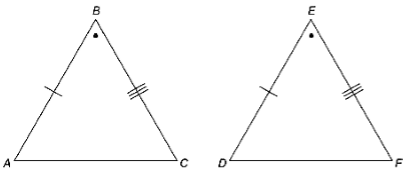If Side AB = DE

Angle ∠B = ∠E

Side BC = EF

Then, ∆ABC ≅ ∆DEF

Example

In ∆JKN, JK = KN and AK is the bisector of ∠JKN, then

1. Find the three pairs of equal parts in triangles JKA and AKN.

2. Is ΔJKA ≅ ΔNKA? Give reasons.

Is ∠J = ∠N? Give reasons.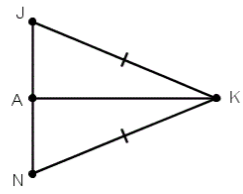Solution

1. The three pairs of equal parts are:

JK = KN (Given)

∠JKA = ∠NKA (KA bisects ∠JKN)

AK = AK (common)

2. Yes, ΔJKA ≅ ΔNKA (By SAS congruence rule)

3. ∠J = ∠N(Corresponding parts of congruent triangles)

3. ASA criterion(Angle-Side-Angle)

This criterion says that the two triangles are congruent if the two adjacent angles and one included side of one triangle are equal to the corresponding angles and one included side of another triangle.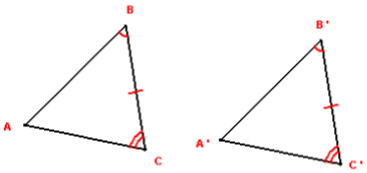If Angle ∠B = ∠B'

Side BC = EF

Angle ∠C = ∠C'

Then, ∆ABC ≅ ∆A'B'C'

Example

In ∆LMN and ∆OPN, if  LMN = ∆NPO = 60°, LNM = 35° and LM = PO = 4 cm.Then check whether the triangle LMN is congruent to triangle PON or not.Solution

In the two triangles ∆LMN and ∆OPN,

Given,

LMN = NPO = 60°

LNM = ∠PNO = 35° (vertically opposite angles)

So, ∠L of ΔLMN = 180° – (60° + 35°)   = 85° (by angle sum property of a triangle) similarly,

∠O of ΔOPN =180° – (60° + 35°) = 85°

Thus, we have ∠L = ∠O, LM = PO and ∠M = ∠P

Now, side LM is between ∠L and ∠M and side PO is between ∠P and ∠O.

Hence, by ASA congruence rule,

∆LMN ≅ ∆OPN.

4. RHS Criterion(Right angle-Hypotenuse –Side)

This criterion says that the two right-angled triangles will be congruent if the hypotenuse and one side of one triangle are equal to the corresponding hypotenuse and one side of another triangle.If Right angle ∠B = ∠E

Hypotenuse AC = DF

Side BC = EF

Then, ∆ABC ≅ ∆DEF

Example

Prove that ∆RSV ≅ ∆RKV, if RS = RK = 7 cm and RV = 5 cm and is perpendicular to SK.Solution

In ∆RSV and ∆RKV,

Given

RS = RK = 7 cm

RV = RV = 5 cm (common side)

If RV is perpendicular to SK then

∠RVS = ∠RVK = 90°.

Hence, ∆RSV ≅ ∆RKV

As in the two right-angled triangles, the length of the hypotenuse and one side of both the sides are equal.

Remark: AAA is not the criterion for the congruent triangles because if all the angles of two triangles are equal then it is not compulsory that their sides are also equal. One of the triangles could be greater in size than the other triangle.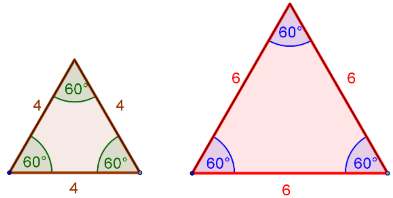In the above figure, the two triangles have equal angles but their length of sides is not equal so they are not congruent triangles.
```### Course Features

• 728 Video Lectures
• Revision Notes
• Previous Year Papers
• Mind Map
• Study Planner
• NCERT Solutions
• Discussion Forum
• Test paper with Video Solution